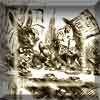#### You may also like### A Problem of Time

Consider a watch face which has identical hands and identical marks for the hours. It is opposite to a mirror. When is the time as read direct and in the mirror exactly the same between 6 and 7?The points P, Q, R and S are the midpoints of the edges of a non-convex quadrilateral.What do you notice about the quadrilateral PQRS and its area?

# Same Length

### Why do this problem?

Geometrical proof is a topic many students find challenging. In this problem, we have brought together two problems about equilateral triangles, so that students can work on the first, discuss it, and develop insights that we hope will give them the tools they need to persevere with the second, harder challenge.

### Possible approach

Show students the animation below, where an equilateral triangle is drawn on two adjacent sides of a square:

"It looks like the triangle formed by the corners is equilateral. How could we prove it?"
Give students some time to discuss ideas. Then bring the class together and share suggestions.
If no-one has suggested drawing a diagram, point out that for most geometry questions it is a really useful first step. They may wish to construct their diagram accurately and measure the three sides; if so, make sure everyone is clear that this does not constitute a proof.

The Key questions below suggest some prompts that could be used to work towards a proof.

Later in the same lesson, or in a follow-up lesson, present the second problem:

For this problem, students may wish to construct several examples to see if it was merely coincidence that the lines were the same length. This could be an opportunity to use a dynamic geometry program like GeoGebra to generate lots of examples. Once students have seen that the sides seem the same length for many examples, challenge them to find a proof. The same prompts in the Key questions below can be used.

Finally, bring the class together and invite students who think they have a proof to present it to the rest of the class. The class could act as "critical friends" to critique each step and make sure the logic is watertight. A possible homework activity could be to write up the steps of the proof clearly.

### Key questions

What angles do you know? (Mark them on your diagram.)
Which lengths are equal? (Mark them on your diagram.)
Which unknown angles are equal? How do you know?
Are there any congruent triangles?

### Possible support

Students may find it helpful to work on some of the Angles, Polygons and Geometrical Proofs short problems before tackling this task.

### Possible extension

For a more challenging example of geometrical proof, see Kite in a Square.